# Original UNIFAC

The Original UNIFAC model consists of the following equations: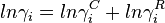$ln \gamma_i = ln \gamma_i^C + ln \gamma_i^R$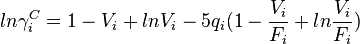$ln \gamma_i^C = 1 - V_i + ln V_i - 5 q_i(1 - \frac{V_i}{F_i} + ln \frac{V_i}{F_i})$

Volume/mole fraction ratio for component i (j: component index) in the mixture: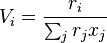$V_i = \frac{r_i}{\sum_j r_j x_j}$

Surface area/mole fraction ratio for component i (j: component index) in the mixture: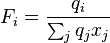$F_i = \frac{q_i}{\sum_j q_j x_j}$

Molecular volume parameter for component i (k: subgroup index):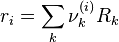$r_i = \sum_k \nu_k^{(i)} R_k$

Molecular surface area parameter for component i (k: subgroup index):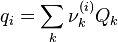$q_i = \sum_k \nu_k^{(i)} Q_k$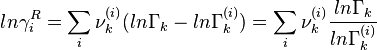$ln \gamma_i^R = \sum_i \nu_k^{(i)} (ln \Gamma_k - ln \Gamma^{(i)}_k ) = \sum_i \nu_k^{(i)} \frac{ln \Gamma_k}{ln \Gamma^{(i)}_k}$

For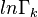$ln \Gamma_k$ group mole fractions (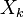$X_k$) and surface area fractions (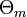$\Theta_m$) are calculated with the composition (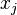$x_j$) of the mixture.

For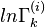$ln \Gamma^{(i)}_k$ group mole fractions (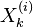$X_k^{(i)}$) and surface area fractions ($\Theta_m$) are calculated with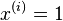$x^{(i)} = 1$ for the pure component i.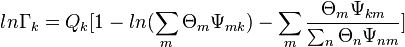$ln \Gamma_k = Q_k [ 1 - ln (\sum_m \Theta_m \Psi_{mk}) - \sum_m \frac{\Theta_m \Psi_{km}}{\sum_n \Theta_n \Psi_{nm}}]$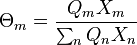$\Theta_m = \frac{Q_m X_m}{\sum_n Q_n X_n}$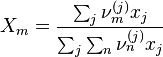$X_m = \frac{\sum_j \nu_m^{(j)} x_j}{\sum_j \sum_n \nu_n^{(j)} x_j}$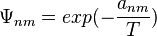$\Psi_{nm} = exp(- { {a_{nm} } \over {T} })$